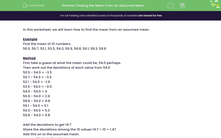# Finding the Mean From an Assumed Mean

In this worksheet, students find the mean from an assumed mean.Key stage:  KS 3

Curriculum topic:   Statistics

Curriculum subtopic:   Understand Variables, Representation, Measures and Spread

Difficulty level:#### Worksheet Overview

In this worksheet, we will learn how to find the mean from an assumed mean.

Example

Find the mean of 10 numbers.
50.5, 50.7, 52.1, 53.5, 54.0, 56.9, 58.8, 59.1, 59.3, 59.8

Method

First take a guess at what the mean could be, 54.0 perhaps.

Then work out the deviations of each value from 54.0

50.5 - 54.0 = -3.5
50.7 - 54.0 = -3.3
52.1 - 54.0 = -1.9
53.5 - 54.0 = -0.5
54.0 - 54.0 = 0
56.9 - 54.0 = 2.9
58.8 - 54.0 = 4.8
59.1 - 54.0 = 5.1
59.3 - 54.0 = 5.3
59.8 - 54.0 = 5.8

Add the deviations to get 14.7

Share the deviations among the 10 values 14.7 ÷ 10 = 1.47

Add this on to the assumed mean.

Mean = 54.0 + 1.47 = 55.47

### What is EdPlace?

We're your National Curriculum aligned online education content provider helping each child succeed in English, maths and science from year 1 to GCSE. With an EdPlace account you’ll be able to track and measure progress, helping each child achieve their best. We build confidence and attainment by personalising each child’s learning at a level that suits them.

Get started